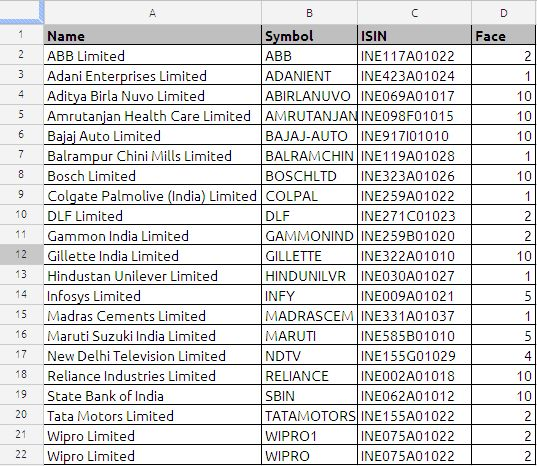## HOW TO SOLVE 3X3 MATRIX USING GOOGLE DOCS

Let say we want to solve the following equations for value of X, Y & Z

3X + 2Y + Z = 15

2X + Y + 3Z = 16

X + 2Y + 3Z = 17

if we convert this in matrix for the problem will look like this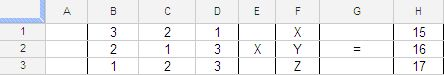We using the two functions namely MINVERSE and MMULT

MINVERSE will calculate the inverse of the matrix and MMULT is used the derive the values of X, Y & Z value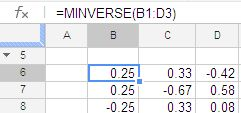the formula in cell B6 is =MINVERSE(B1:D3)

which is nothing but the inverse of the matrix

now to solve the X, Y, Z we are using the MMULT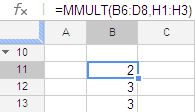the formula in cell B11 is =MMULT(B6:D8,H1:H3)

so the solution to the problem is X=2, Y=3 & Z=3

you can also use the both in the same formula which will be =MMULT(MINVERSE(B1:D3),H1:H3)

## ASCII Character set in Google Docs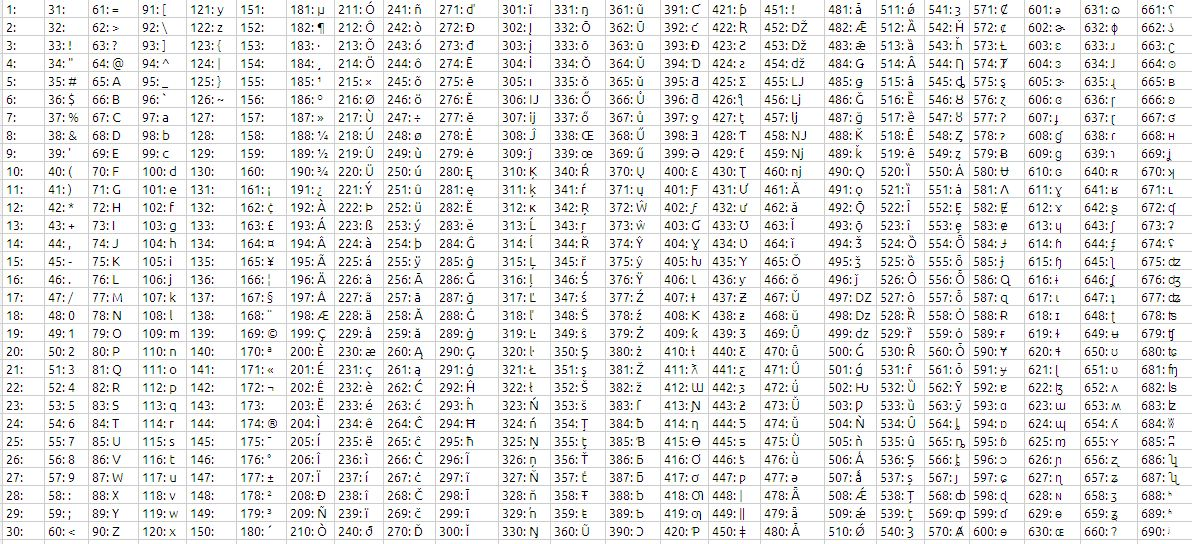The formula used to generate this character set is as follows:

=ARRAYFORMULA(ROW(1:30)+(COLUMN(A:AC)*30-30)&”: “&CHAR(ROW(1:30)+(COLUMN(A:AC)*30-30)))

For each Column you will have 30 characters

the format in each cell is number followed by ASCII character

## Net Present Value or NPV

The NPV function returns the net present value of an investment based on a series of potentially irregular cash flows that occur at regular time intervals.

Oxford Dictionary of Accounting defines Net Present Value(NPV) as

“A method of capital budgeting in which the value of an investment is calculated as the total present value of all cash inflows and cash outflows minus the cost of initial investment. If the net present value is positive, the return will be greater than that required by the capital markets and investment should be considered. A negative net present value indicates that the investment should be rejected.”

Example:

A Company is considering the purchase of a new machine, which is expected to save 100,000 in cash operating costs each year. Its estimated useful life(how long it will last) is five years, at the end of which it will have no net residual value.

The cash flows for the project are as follows:

Year 0 : Cost of the machine 390,000

Year 1: Savings of 100,000

Year 2: Savings of 100,000

Year 3: Savings of 100,000

Year 4: Savings of 100,000

Year 5: Savings of 100,000

A simple analysis shows that the savings over five years are 500,000 (5 X 100,000) and the cost is 390,000, which gives a surplus of 110,000. However, such an analysis ignores the time value of money; 1 received in future is not equal to 1 received today. To calculate the present value of future cash flows it is necessary to calculate the discount factor.

Assuming that the cost of capital for the project is 8%, the discount factor is calculated as follows:

 Year 1 1/(1.08)^1 Year 2 1/(1.08)^2 Year 3 1/(1.08)^3 Year 4 1/(1.08)^4 Year 5 1/(1.08)^5

The net present value can then be calculated: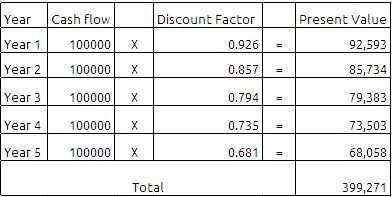If we deduct the initial investment of the machine 390,000 then we will get 9,271 which is positive. so we can accept the investment in the project since it is giving the positive cash flow.

All the above is good to understand the background of the Net Present Value (NPV) as far as finance is concerned.

Now we will see how to use this function in google docs spreadsheets.

The Syntax of the function is as follows:

NPV(Rate, value_1, value_2, … value_30)

Rate: The discount rate per period. periodic-discount-rate is a number value and is either entered as a decimal (for example, 0.08) or with a percent sign (for example, 8%). periodic-discount-rate must be greater than or equal to 0.

Value_1, Value_2, …….Value_3 :Values is a number value. A positive value represents income (cash inflow). A negative value represents an expenditure (cash outflow). Value must be equally spaced in time.

Value_1………..: Optionally include one or more additional cash flows.

Usage Notes

periodic-discount-rate is specified using the same time frame as the time frame used for the cash flows. For example, if the cash flows are monthly and the desired annual discount rate is 8%, periodic-discount-rate must be specified as 0.00667 or 0.667% (0.08 divided by 12).

If cash flows are irregular, use the IRR function.

We can achieve the same results using the NPV function as shown in the below image: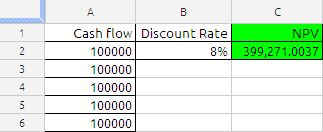The formula in cell C2 is

=NPV(B2,A2:A6)

## VLOOKUP

Vlookup is one of the most powerful functions in Spread sheets. So many people use Vlookup as a daily function, and is most useful in searching a table, looking for the same names, field or identifier and then spitting out an output based on that search criteria. It may sound difficult, but is quite easy

The VLOOKUP function retrieves an item from a table and returns it to a cell or formula.

The Syntax of the function is

VLOOKUP(search_criterion, array, index, sort_order)

The VLOOKUP:

1) Looks for a value in the leftmost column of a table

2) And then returns a value in the same row from a column you specify

3) By default, the table must be sorted in an ascending order

The VLOOKUP function has four arguments:

1) search_criterion: This is the items that the VLOOKUP looks at before it goes over to the table and looks it up. Once the VLOOKUP goes over to the table, it looks in the leftmost column to try and fine the value

2) array : is the table (database) that you are looking a value up in

3) index : is the number of the column that has the value you want to get and return back to the cell (the leftmost column of the table is considered 1). For this argument you can use the COLUMNS or ROWS functions to increment numbers.

4) sort_order : is a 0 when you are looking up an exact value (like a word) and 1 or omitted when you are looking up an approximate value (like in tax tables where you want to find the row that is equal to the lookup_value or greater than, but less than the next value in the leftmost column.

Notes:

• If index is less than 1, the VLookup function will return #VALUE!.
• If index is greater than the number of columns in table_array, the VLookup function will return #REF!.
• If you enter FALSE for the not_exact_match parameter and no exact match is found, then the VLookup function will return #N/A.

(1) The search_criterion argument is the “number that we are looking up.”.

• NOT case sensitive (TOM=tom=ToM=tOM)
• Cannot be longer than 255 characters.
• Can be a number, text, logical value, or a name or reference that refers to a one of these

(2) The array argument is the lookup table and can contain one or more columns.

• The first column is the lookup column
• The column with the values to return can be in any of the columns(1,2,3, and so on)
• If you are doing an approximate match, the first column must be sorted smallest to biggest.
• If you are doing an exact match, the first column does not need to be sorted.

(3) The Index argument is a number (1,2,3, and so on) that represented the column in the table_array that has the value to return to the cell.

(4) The sort_order argument tells the VLOOKUP function whether you are doing an approximate match or an exact match or an exact match when looking up a value.

If you are doing an approximate match, the first column must be sorted smallest to biggest(ascending)

If there are duplicates in the first column, VLOOKUP will choose the first one listed.

Now let see and understand the working of VLOOKUP function:

The sample data is taken from NSE (National Stock Exchange) of India web site, the first column is name of the company, second column in NSE stock symbol, third column is ISIN number and the  fourth column is face value of stock script.

the data used in this example available at the end of the post

suppose we want stock symbol of Gillette India Limited in cell B5 the formula will be

= VLOOKUP(A24,A2:D21,2,FALSE)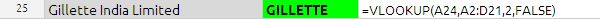in the above example search_criterion argument is A24 it is name of the stock we want to search in the data base or table.

array argument is table array which is from A2 to D21

index argument is the column index number in the above example it is  2 which stock symbol corresponding to script.

sort_order in the above example sort_order is FALSE because we want to exact match.

If there is no match for the search_criterion, the VLOOKUP spit the data one row above the nearest value.In the above example the APPLE COMPUTERS INC does not have any value in the table, but since we have put the Sort_order as TRUE which is nothing but approximate match. we have got the AMRUTANJAN.

In case of duplicate values the VLOOKUP function return the value in the first place.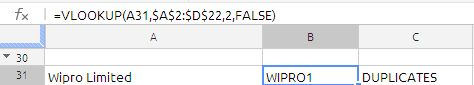in the data table if you see A21 and A22 has the same value, VLOOKUP function will return first value only. this is big constrain in the  VLOOKUP function.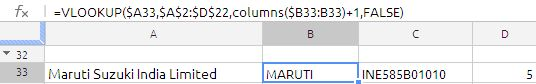the formula in cell B33 is

=VLOOKUP(\$A33,\$A\$2:\$D\$22,COLUMNS(\$B33:B33)+1,FALSE

If we drag it across we will get the data from  Symbol,ISIN, Face. the only logic inside the formula is correct use of relative reference and use of COLUMNS function which is column increment in the  above formula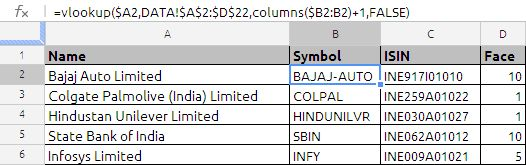in the above example the formula used in cell B2 is =VLOOKUP(\$A2,DATA!\$A\$2:\$D\$22,COLUMNS(\$B2:B2)+1,FALSE) drag it down and   cross

the above formula will fill the column B, C & D.

the above formula is traditional way of writing. But same can be modified in the google docs array formula as shown in the below example: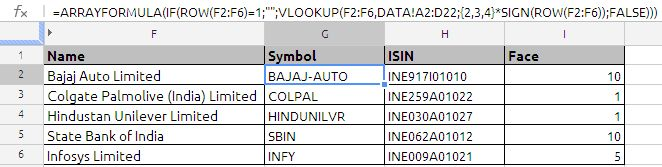this is single most efficient, no dragging is required.

the formula used in cell G2 is =ARRAYFORMULA(IF(ROW(F2:F6)=1;””;VLOOKUP(F2:F6,DATA!A2:D22;{2,3,4}*SIGN(ROW(F2:F6));FALSE)))

most noticeable point is use of {2,3,4} which is array of column numbers which we want to extract. SIGN function is used to convert the true /false to 1 and 0

VLOOKUP is more versatile, most used function, i am only trying to explain with basic futures only.

.